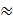### Sample Problem

You are going to San Francisco to visit your Aunt Carly. On the way there, the plane is flying into a headwind; your flight from Boston, Massachusetts to San Francisco, California takes 6 hours and 15 minutes. However, on the return flight, this same 3000 mile distance between the two cities is covered in 5 hours and 30 minutes. Find the airspeed of the plane and the speed of the wind, assuming that both remain constant.

Speed of plane = mph

Speed of wind = mph

Round all answers to one decimal place.

#### Solution

The two unknown quantities are the speeds of the wind and the plane.

If s1=speed of the plane and s2=speed of the wind, then

s1-s2= speed of the plane against the wind

s1+s2=speed of the plane with the windUsing the formula distance=(rate)(time) for these two speeds, you get the following equations

3000=(s1-s2)(6+15/60) , 3000=6.25s1-6.25s2,12000=25s1-25s2

3000=(s1+s2)(5+30/60), 3000=5.5s1+5.5s2 , 6000=11s1+11s2Using elimination to solve this system, multiply the first equation by 11 and the second equation by 25.

132000=275s1-275s2

+(150000=275s1+275s2)

282000=550s1+0

S1=5640/11512.7 mph

By back substituting s1=5640/11 mph into either equation,

6000=11(5640/11)+11s2

360/11=s232.7 mph

S1512.7 mph ; s232.7 mph